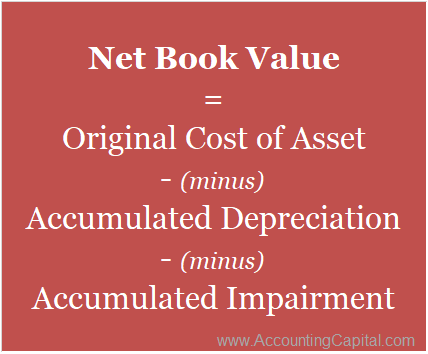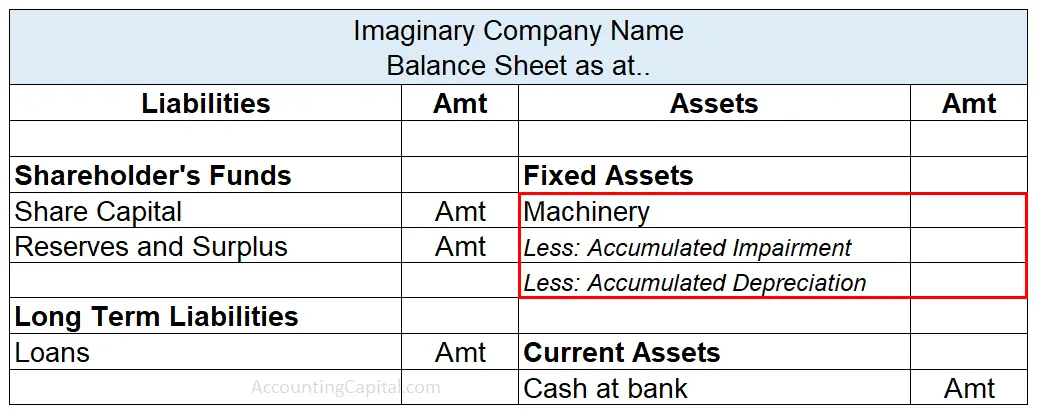# What is Net Book Value?

## Meaning and Formula

Net Book Value represents the carrying value of an asset that is equal to the value after deducting depreciation, depletion, amortization and/or accumulated impairment, to date. It is the value at which an asset is recorded in the balance sheet of an enterprise.

Further, normal wear-tear, obsolescence, natural factors and other such factors lead to a fall in the value of an asset over the course of its expected useful life. Such a loss is termed as;

• Depreciation – for tangible fixed assets
• Depletion – for natural resources
• Amortization – for intangible assets

However, impairment involves an unexpected and extraordinary drop in the value of an asset.

Net Book Value helps in reflecting the value of an unutilized asset as on a given date because of which, it is also termed as Net Asset Value or Carrying Value.

Formula to Compute Net Book Value / Net Asset ValueNote – When an asset reaches the end of its expected useful life, its net book value equals its salvage value.

Related TopicIs accumulated depreciation an asset or liability?

## How to Calculate Net Book Value

Steps to Calculate N.B.V of an Asset

Step 1 – Find the historical cost of the asset by computing its total cost of acquisition.

Step 2 – Calculate the total amount of depreciation to be charged on the asset to date.

Step 3 – Subtract accumulated depreciation from the historical cost of the asset.

Example 1 – Suppose a company purchases a pre-owned truck worth 80,000 & further, incurs a cost of 10,000 for its repairs before using it. Also, it decides to charge depreciation @ 10% as per the straight-line method.

Original cost of truck = cost of acquisition + cost of repairs

= 80,000 + 10,000 = 90,000

Depreciation after first year = 90,000 x 10% = 9,000

Net Asset Value of the truck will be as follows,

• Year 1 = 90,000 – 9,000 = 81,000
• Year 2 = 81,000 – 9,000 = 72,000
• Year 3 = 72,000 – 9,000 = 63,000
• Year 4 = 63,000 – 9,000 = 54,000

and so on.

Example 2 – With accumulated depreciation, impairment loss and salvage value.

Suppose VIP Ltd. purchased machinery worth 2,00,000, with a useful life of 10 years. Its salvage value at the end to 10 years was estimated to be 10,000. After 2 years, the company revalued the asset and its revised value at the end of the 2nd year turns out to be 1,40,000. Its remaining useful life was re-estimated at 5 years with nil salvage value. The company uses the straight-line method of depreciation.

We know, Depreciation = (Cost of asset – Salvage value) / Useful Life

Initial Depreciation per year = (2,00,000 – 10,000) / 10 = 1,90,000 / 10 = 19,000

At the end of 2nd year

Accumulated Depreciation = 19,000 X 2 = 38,000

Net book value = Cost of Asset – Accumulated depreciation

= 2,00,000 – 38,000 = 1,62,000

Calculation of Impairment Loss

Impairment loss = Revalued Value – Carrying value of the asset

= 1,40,000 – 1,62,000 = 22,000

Calculation of new Depreciation for machinery (for 3rd year onwards)

Depreciation per year = (Revised Value of asset – Revised Salvage value) / Revised Life

= (1,40,000 – 0) / 5 = 28,000

Note: Impairment loss of 22,000 will be debited to the profit and loss account. Machinery will be shown at its revised value, which is 1,40,000, at the end of 2nd year. This can also be verified with the formula as follows;

Net Book Value = Original Cost – Accumulated depreciation – Impairment loss

= 2,00,000 – 38,000 – 22,000 = 1,40,000

Related TopicAre accounts receivable assets or revenue?

## NBV in Financial Statements

The term Net Book Value (NBV) is related to the net value of a firm’s assets & hence, it is shown on the asset side of a balance sheet.## Net Book Value Vs. Book Value Vs. Market Value

Both Net Book Value & Book Value simply refer to the value of unused assets left with the organization. They are both equal to the difference between the historical cost of an asset and the amount of depreciation/impairment accumulated on that. Therefore, they are quite synonymous and may be used interchangeably.

Market Value is the amount that an asset will bring if it is sold in the market today. It is the price that people are willing to pay in an open market for an asset. Any asset’s market value and book value are usually never the same.

For example, a business that owns a laptop for 50,000 lasts for five years. The depreciation charged is at 20% every year. After the end of the 1st year, its net book value (or book value) will be 50,000 – 20%, i.e. 40,000.

However, if the business decides to sell the same laptop in an open market after 1 year it might only fetch 20,000. The maximum amount a buyer is willing to pay for the laptop after one year is its market value.

Recording Assets at their Net book value is of high importance because of the following reasons;

1. It helps in maintaining more accurate accounting records because the value of assets is recorded after deducting any actual or anticipated loss as per prudence.
2. It helps in estimating the real value of the assets which also helps in business valuation at the time of liquidation of the company.

Using Net Book Value can be misleading because of the following two disadvantages;

1. NBV is sometimes mistaken as market value however, as explained earlier, it is never equal to the market value of the asset. Market value is the amount that an asset will get if it is sold in the market. Many times, the market value of an asset is too less than its book value because of which, book value can show an inaccurate image of the position of a company.
2. The estimates made by the management has an impact on the net book value of assets. For example, the rate of depreciation and the scrap values of an asset has a direct effect on its NBV.